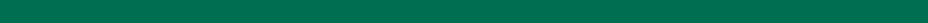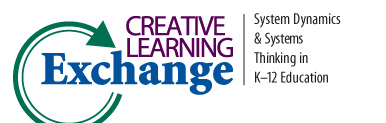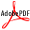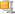>
CLE Curriculum Search
Search results for: Math
116 records found. Currently displaying page 5 of 12 [<< Prev] 1 2 3 4 5 6 7 8 9 10 11 12 [Next >>]
 Lessons in Mathematics Section 4: Review Author(s): Diana M. Fisher Subject: Math This book provides a set of tools that enables educators to teach mathematics using the framework of System Dynamics. Section 4 reviews previous concepts, combines model structures to solve new problems and extends problems from previous lessons. Availab More about the book at: http://www.iseesystems.com/store/college_university/MathBook.aspx Lessons in Mathematics Section 5: Oscillatory Behavior Author(s): Diana M. Fisher Subject: Math This book provides a set of tools that enables educators to teach mathematics using the framework of System Dynamics. Section 5 covers sinusoidal functions, including simple harmonic motion. Distance versus time lessons using a motion detector are also inPDFMore about the book at: http://www.iseesystems.com/store/college_university/MathBook.aspx Lessons in Mathematics Section 6: Convergent and Logistic Behavior Author(s): Diana M. Fisher Subject: Math This book provides a set of tools that enables educators to teach mathematics using the framework of System Dynamics. Section 6 takes students through the steps from exponential to convergent to logistic models, culminating with an application to a systemPDFMore about the book at: http://www.iseesystems.com/store/college_university/MathBook.aspx Lessons in Mathematics Section 7: Differential Equations Author(s): Diana M. Fisher Subject: Math This book provides a set of tools that enables educators to teach mathematics using the framework of System Dynamics. Section 7 builds on the skills of Sections 5 and 6 using classic problems studied with differential equations. Exponential, convergent anPDFMore about the book at: http://www.iseesystems.com/store/college_university/MathBook.aspx Lessons in Mathematics Section 8: Miscellaneous Topics Author(s): Diana M. Fisher Subject: Math This book provides a set of tools that enables educators to teach mathematics using the framework of System Dynamics. Section 8 presents a natural extension of linear and exponential growth study - arithmetic and geometric sequences. Application to an age More about the book at: http://www.iseesystems.com/store/college_university/MathBook.aspx Lessons in Mathematics Section 9: Appendix Author(s): Diana M. Fisher Subject: Math This book provides a set of tools that enables educators to teach mathematics using the framework of System Dynamics. Section 9 is a useful reference for all lessons in the book. Included are generic modeling structures, a summary of motion, distance-velo More about the book at: http://www.iseesystems.com/store/college_university/MathBook.aspx Lessons in Mathematics: A Dynamic Approach Author(s): Diana M. Fisher Subject: Math The abstractness of a traditional approach to mathematics causes many students to falter. This book focuses on making the abstractness more concrete with interesting and fun lessons. It uses STELLA software's icon-based, non-abstract language to structure More about the book at: http://www.iseesystems.com/store/college_university/MathBook.aspx Let It Roll! An Interdisciplinary Middle School Math/Science Unit Using a STELLA Model of the Physics of Motion Author(s): Rob Quaden, J. Trierweiler, & D. Lyneis Subject: Cross-Curricular In this unit eighth graders first conduct a physics of motion laboratory experiment and then try to understand the behavior using model building, math reasoning and scientific reasoning.Zipped (Models & PDF) Linear Models in STELLA Author(s): Diana M. Fisher, & Rogers Subject: Math From CC-STADUS. Introductory tutorial exercises using STELLA II for high school math. Involves distance, car depreciation and jumping out of an airplane.Zipped (Models & PDF) Linear Systems Using STELLA Author(s): Diana M. Fisher Subject: Math From CC-STADUS. Semi-complex STELLA tutorial. Involves train robbery, Star Trek, and camping.PDF
Sorted Ascending by Title Hide Descriptions